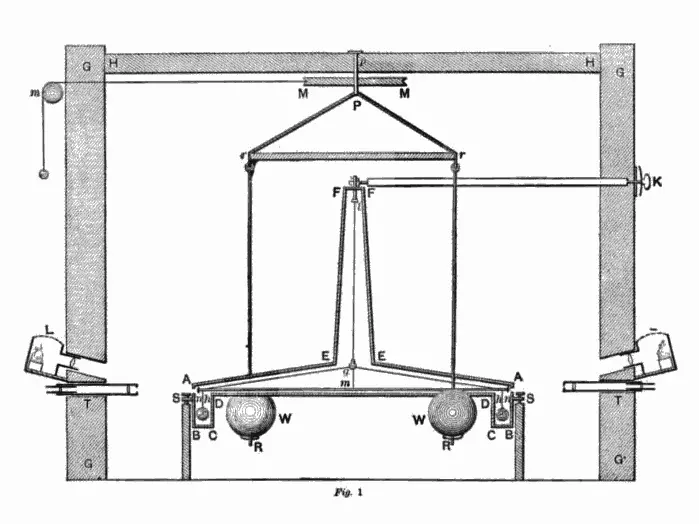## 高等数学物理应用：质点间引力的计算公式图 02. 引力常量 $G$ 最先由英国物理学家卡文迪许测量得出，如图所示为卡文迪什对应的论文中的第一张图片，描述了测量引力常数的扭秤实验的结构。创作时间：1798 年 06 月 21 日。Public domain.

## 一、前言$$\textcolor{orangered}{ \cos (\arcsin x) = ? }$$

$$\textcolor{springgreen}{ \sin (\arccos x)= ? }$$

## 一、前言$$\sin (\arctan x) = ?$$

$$\cos (\arctan x) = ?$$

## 一、前言$$\frac{\mathrm{d} y}{\mathrm{d} x} = \frac{1}{\frac{\mathrm{d} x}{\mathrm{d} y}}$$

## 一、前言$$a^{2} + b^{2} \geqslant 2ab$$

$$a + b \geqslant 2 \sqrt{ab}$$

Tips:

## 一、前言豫公网安备41142502000132号